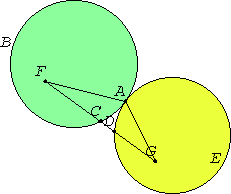Proposition 12

If two circles touch one another externally, then the straight line joining their centers passes through the point of contact.
III.1

Let the two circles ABC and ADE touch one another externally at the point A. Take the center F of ABC, and the center G of ADE.I say that the straight line joined from F to G passes through the point of contact at A.

For suppose it does not, but, if possible, let it pass as FCDG. Join AF and AG.

Then, since the point F is the center of the circle ABC, FA equals FC.

Again, since the point G is the center of the circle ADE, GA equals GD.

I.20

But FA was also proved equal to FC, therefore FA and AG equal FC and GD, so that the whole FG is greater than FA and AG, but it is also less, which is impossible.

Therefore the straight line joined from F to G does not fail to pass through the point of contact at A, therefore it passes through it.

Therefore if two circles touch one another externally, then the straight line joining their centers passes through the point of contact.

Q.E.D.

Guide

This proposition was probably added to the Elements after Euclid, perhaps by Heron or a later commentator.

This proposition is not used in the rest of the Elements.